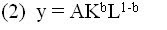## Sunday, September 23, 2007

### A Brief Look at the Productivity Norm Rule

Mark Toma points us to knzn who is arguing the Fed should target unit labor costs. I am sympathetic to this view because it would mean--depending on how it was implemented--monetary policy would allow productivity-driven deflation. Currently, all deflationary pressures are banished by monetary authorities and I believe this approach--which fails to distinguish between aggregate demand-driven or malign deflation and aggregate supply or benign deflation--is destabilizing to the macroeconomy (see here and here for reasons why). In fact, I have argued that this one-size-fits-all approach to deflationary pressures contributed to the housing boom of 2003-2005.

What I have not done is spell out how one could systematically allow for benign deflationary pressures in monetary policy while correcting for malign deflationary pressures. That is why knzn's proposal is so interesting to me. His ideas line up nicely with George Selgin's work, which shows how to implement a monetary policy rule that allows for benign deflation. Selgin proposes a "Productivity Norm" rule that in one form would effectively stabilize the nominal wage but allow the price level to reflect changes in productivity.

There are actually two forms of his rule. Under the first one--the "Total Factor Productivity Norm" rule--Selgin would have monetary authorities target a nominal income growth rate equal to the expected growth rate of real factor inputs. Such a nominal income target would monetarily accommodate the real output effect of factor input growth, but not productivity growth and therefore allow the price level to inversely reflect both shocks to and anticipated changes in productivity. Under the second version--the "Labor Productivity Norm" rule-- Selgin would have nominal income growing at the expected growth rate of labor inputs. This monetary policy rule would still stabilize the nominal wage but lead to a slightly higher rate of deflation than the total factor productivity norm rule. These productivity norm rules, like other nominal income stabilizing rules, would also provide a natural offset against aggregate demand shocks, thus correcting for malign deflationary pressures.

The productivity norm rules are intended to improve macroeconomic stability by allowing for benign deflation. Why benign deflation is important to macroeconomic stability has been outlined in this blog (see above links), but to see Selgin's reasons look at his book "Less than Zero: the Case for a Falling Price Level in a Growing Economy" or this paper.

Below I have copied (with some slight adjustments) from Selgin's above book the appendix that outlines in a more formal way how the two productivity norm rules would appear in nominal income targeting rules.

"Let

(1) Py = wL+rK

represent an economy's nominal income, where P is the general price level, y is real output, w is the price of a unit of average-quality labor, r is the rental price of average-quality capital, L is labor input and K is capital input. Also, letbe the economy's productivion function, where A is a total factor productivity index and b is capital's share of total income, rK/Py, which is assumed to be constant (as is roughly the case in reality). The logarithmic differential of (2) with respect to time is:

(3) y = A + bK +(1-b)L,

where italics represent growth rates. A, then, is the growth rate of total factor productivity. Rearranging (3) gives

(4) y - L = A + b(K-L)

where y-L is the growth rate of labor productivity and (K-L) is the growth rate of the capital-labor ratio. A labor productivity norm requires that

(5) P = L - y

whereas a total factor productivity norm requires that P = -A or equivalently, (from equation 4) that

(6) P = -y + bK + (1-b)L

Equations (5) and (6) can be rearranged to give corresponding rules for nominal income growth. A labor productivity norm requires that

(7) P + y = L,

that is, that nominal income grow at the same rate as labor input; while a total factor productivity norm requires that

(8) P + y = bK + (1-b)L,

that is, that nominal income grow at a rate equal to a weighted average of the growth rates of labor and capital input.

Lastly, we can compare the behaviour of (constant-quality) money wages under the two regimes by taking the logarithmic differential of (1) and recalling that b=rK/Py = a constant:

(9) P + y = w + L.

By substituting (7) and (8), respectively into (9), and solving in each case for w, we find that, under a labor productivity norm,

w = 0

meaning that money wages are kept stable; whereas, under a total factor productivity norm,

w = b(K-L),

meaning that money wages rise as production becomes more capital intense."